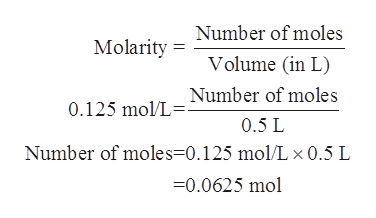# What mass of lithium phosphate is needed to prepare 500. mL of a solution having a lithium ion concentration of 0.125 M?

Question
601 views

What mass of lithium phosphate is needed to prepare 500. mL of a solution having a lithium ion concentration of 0.125 M?

check_circle

Step 1

The number of moles for a given chemical substance are defined as a ratio of its mass to that of molar mass. The mathematical formula is as follows:

Step 2

The concentration or the molarity for a given solution is defined as the ratio of number of moles of solute to its volume. The SI units of molarity are moles/L. It is denoted by the symbol ‘M’. The mathematical relationship is expressed as follows:

Step 3

The concentration of the lithium phosphate solution is given to be 0.125 M.

The volume of the solution which is needed to be prep...help_outlineImage TranscriptioncloseNumber of moles Molarity Volume (in L) Number of moles 0.125 mol/L= 0.5 L Number of moles=0.125 mol/L x 0.5 L -0.0625 mol fullscreen

### Want to see the full answer?

See Solution

#### Want to see this answer and more?

Solutions are written by subject experts who are available 24/7. Questions are typically answered within 1 hour.*

See Solution
*Response times may vary by subject and question.
Tagged in
ScienceChemistry

### Physical Chemistry